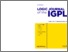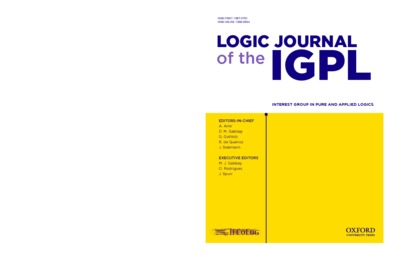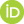# Logical Information Theory: New Logical Foundations for Information Theory

Ellerman, David (2017) Logical Information Theory: New Logical Foundations for Information Theory. [Preprint]Preview
Text
Logic-to-information-theory3.pdf

## Abstract

here is a new theory of information based on logic. The definition of Shannon entropy as well as the notions on joint, conditional, and mutual entropy as defined by Shannon can all be derived by a uniform transformation from the corresponding formulas of logical information theory. Information is first defined in terms of sets of distinctions without using any probability measure. When a probability measure is introduced, the logical entropies are simply the values of the (product) probability measure on the sets of distinctions. The compound notions of joint, conditional, and mutual entropies are obtained as the values of the measure, respectively, on the union, difference, and intersection of the sets of distinctions. These compound notions of logical entropy satisfy the usual Venn diagram relationships (e.g., inclusion-exclusion formulas) since they are values of a measure (in the sense of measure theory). The uniform transformation into the formulas for Shannon entropy is linear so it explains the long-noted fact that the Shannon formulas satisfy the Venn diagram relations--as an analogy or mnemonic--since Shannon entropy is not a measure (in the sense of measure theory) on a given set.
What is the logic that gives rise to logical information theory? Partitions are dual (in a category-theoretic sense) to subsets, and the logic of partitions was recently developed in a dual/parallel relationship to the Boolean logic of subsets (the latter being usually mis-specified as the special case of "propositional logic"). Boole developed logical probability theory as the normalized counting measure on subsets. Similarly the normalized counting measure on partitions is logical entropy--when the partitions are represented as the set of distinctions that is the complement to the equivalence relation for the partition.
In this manner, logical information theory provides the set-theoretic and measure-theoretic foundations for information theory. The Shannon theory is then derived by the transformation that replaces the counting of distinctions with the counting of the number of binary partitions (bits) it takes, on average, to make the same distinctions by uniquely encoding the distinct elements--which is why the Shannon theory perfectly dovetails into coding and communications theory.

 Export/Citation: EndNote | BibTeX | Dublin Core | ASCII/Text Citation (Chicago) | HTML Citation | OpenURL
 Social Networking:

Item Type: Preprint
Creators:
CreatorsEmailORCIDEllerman, Daviddavid@ellerman.org
Keywords: partition logic, logical entropy, Shannon entropy
Subjects: Specific Sciences > Mathematics > Logic
Specific Sciences > Computation/Information
Depositing User: David Ellerman
Date Deposited: 17 Jul 2017 15:56
Item ID: 13213
Subjects: Specific Sciences > Mathematics > Logic
Specific Sciences > Computation/Information
Date: 2017
URI: http://philsci-archive.pitt.edu/id/eprint/13213

### Actions (login required)View Item